Application

# How Does an IMU Work?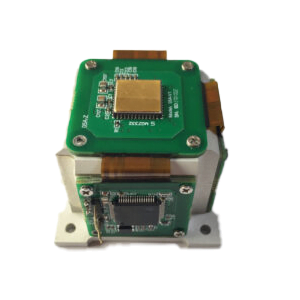Ⅰ.IMU introduction

IMU (Inertial measurement unit) is a device that measures the three-axis attitude angle and acceleration of an object. Generally, IMUs include a three-axis gyroscope and a three-axis accelerometer, and some 9-axis IMUs also include a three-axis magnetometer.The three-axis acceleration sensor works based on the basic principle of acceleration.

Three-axis accelerometer principle

The three-axis accelerometer is an inertial sensor that can measure the specific force of an object, that is, the overall acceleration after removing gravity or the non-gravitational force acting on unit mass. When the accelerometer remains stationary, the accelerometer senses the acceleration due to gravity, and the overall acceleration is zero. In free fall, the overall acceleration is the acceleration of gravity, but the accelerometer is in a weightless state inside, and the output of the three-axis accelerometer is zero at this time.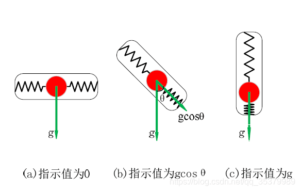The principle of a three-axis accelerometer can be used to measure angles. Intuitively, as shown in the figure, the amount of spring compression is determined by the angle of the accelerometer to the ground. Specific force can be measured by the compression length of the spring. Therefore, in the absence of external force, the accelerometer can accurately measure pitch and roll angles without cumulative error.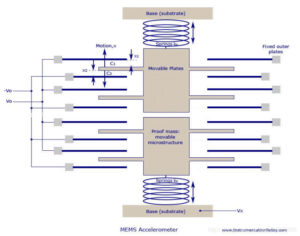MEMS three-axis accelerometers use piezoresistive, piezoelectric and capacitive working principles. The specific force (pressure or displacement) generated is proportional to changes in resistance, voltage and capacitance respectively. These changes can be collected through corresponding amplification and filtering circuits. The disadvantage of this sensor is that it is greatly affected by vibration. Three-axis accelerometers cannot measure yaw angle due to their working principle of measuring angle: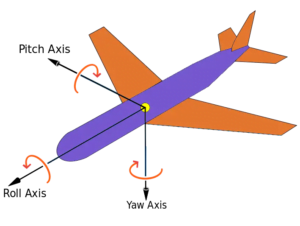Three-axis gyroscope principle

The three-axis gyroscope is the core sensitive device of the inertial navigation system, and its measurement accuracy directly affects the accuracy of the attitude calculation of the inertial navigation system. Function: Used to measure the angular velocity in the unit and the calculation of the angle after integrating the angular velocity. The Coriolis force is also called the Coriolis force in some places, or Coriolis force for short. It is a straight line in a rotating system. A description of the deflection of a moving particle due to inertia relative to the linear motion produced by the rotating system. The Coriolis force comes from the inertia of an object’s motion. When a particle moves in a straight line relative to the inertial system, its trajectory is a curve relative to the rotating system due to its own inertia. Based on the rotation system, we believe that there is a force driving the movement trajectory of the particle to form a curve. The Coriolis force is a description of this shift and is expressed as: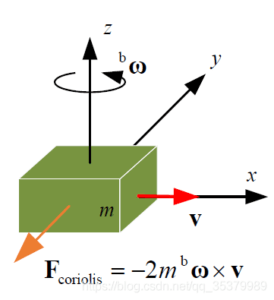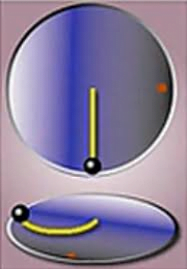That is to say, the linear motion of the original linear motion will deviate when placed in a rotating system, but in fact the linear motion is not affected by a force. The establishment of such a virtual force is called the Coriolis force.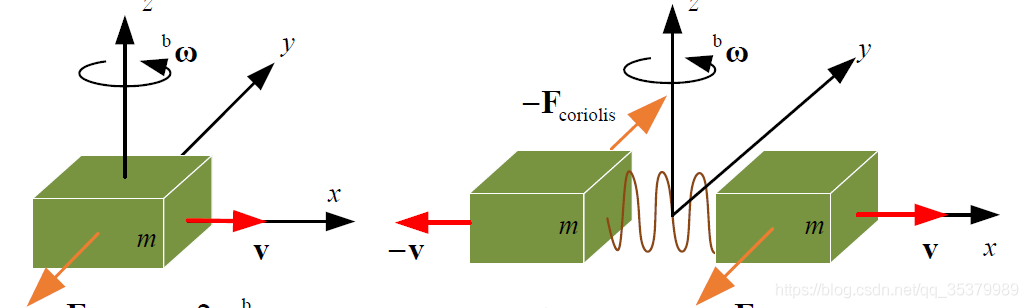Therefore, we select two objects in the gyroscope. They are in constant motion, and the phase difference of their movements is -180 degrees. That is, the two mass blocks move in opposite directions but have the same size. The Coriolis forces they generate are opposite, thus forcing the two corresponding capacitor plates to move, resulting in a differential change in capacitance. The change in capacitance is proportional to the angular velocity of rotation. The change in rotation angle can be obtained from the capacitance.

Magnetometer principle

The magnetometer can provide data on the magnetic field experienced by the device in each of the XYZ axes. The relevant data will then be fed into the algorithm of the microcontroller to provide the heading angle related to the magnetic north pole. This information can be used to detect geographical orientation.

The magnetometer uses three mutually perpendicular magnetoresistive sensors. The sensor in each axis detects the strength of the geomagnetic field in that direction.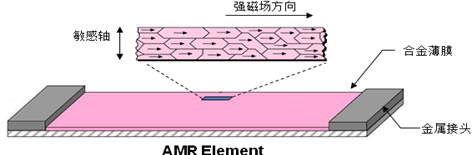The picture above shows an alloy material with a crystal structure. They are very sensitive to external magnetic fields, and changes in the strength of the magnetic field will cause changes in the resistance value of the magnetoresistive sensor.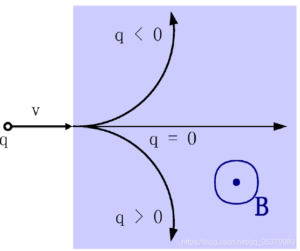In addition, the three-axis magnetometer can also use the Lorentz force principle. The current flows through the magnetic field to generate force, thereby driving changes in capacitance and so on.

Ⅱ.What sensors are inside the IMU and how do they work?

Inertial measurement units typically consist of three different types of sensors. The first type of sensor is an accelerometer, which measures acceleration, or the rate at which an object accelerates or decelerates. While there are many different sensor technologies for accelerometers, by far the most common for wearable applications is MEMS (microelectromechanical systems). MEMS are sensor systems composed of electrical and mechanical components, typically etched from micron-sized silicon.

Whenever the MEMS accelerometer experiences acceleration, the proof mass also experiences that acceleration. An etched spring set resists this acceleration. Using Hooke’s law (spring force is proportional to the distance the spring is compressed) and Newton’s second law (force is proportional to acceleration), check that the distance a mass moves is proportional to the acceleration it experiences (see figure below). This movement is sensed using the electrical properties of capacitance, which is related to the distance between two conductors. A set of electronics is then able to measure the change in capacitance, calibrate the signal, and further process it to give acceleration.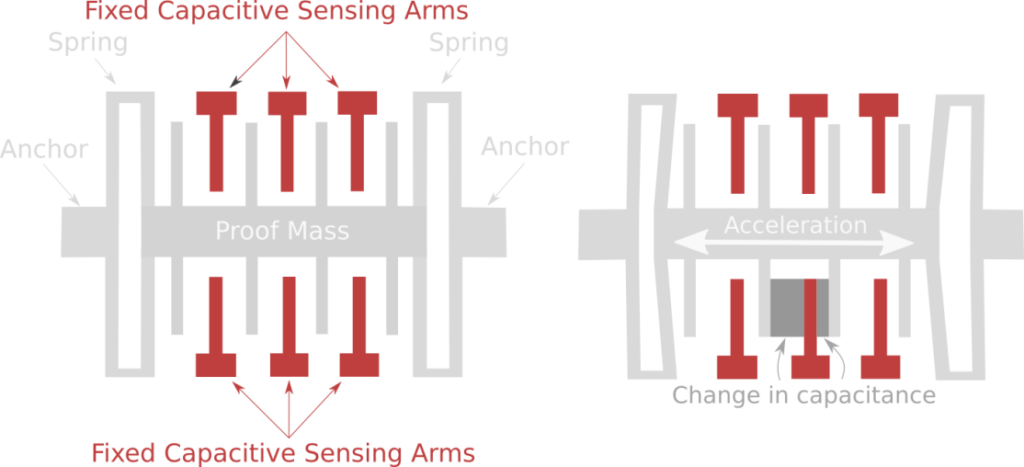The second type of sensor in an Inertial measurement unitis a gyroscope, which measures angular velocity, or the speed and direction of an object’s rotation or spin. Gyroscopes also typically use MEMS technology, although they are more complex than MEMS accelerometers. The main physical phenomenon used in gyroscopes is the Coriolis effect, which describes the forces involved when an object moves in a rotating reference frame.

MEMS gyroscopes have masses that reciprocate at a constant frequency. During the rotation of the gyroscope, due to the Coriolis effect, the mass will induce a force perpendicular to the direction of the reciprocating motion. This force is counteracted by an etched spring and sensed by a capacitive sensing arm such as an accelerometer. Signal processing electronics then process the change in capacitance relative to the reciprocating motion of the resonant mass (see figure below).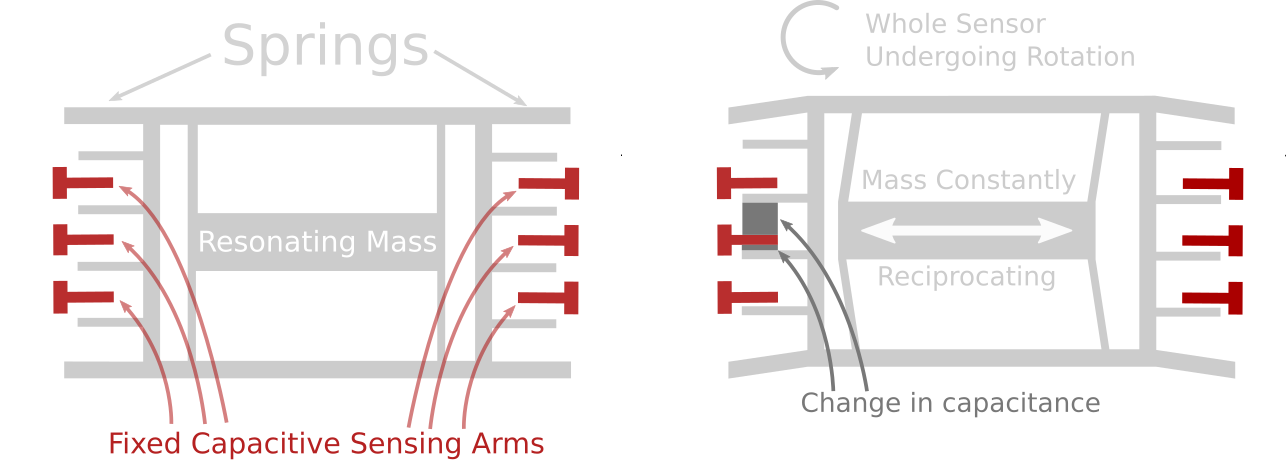The final sensor commonly found in Inertial measurement units is a magnetometer, which measures the strength of a magnetic field and acts somewhat like a digital compass. Most magnetometers use the Hall effect to measure magnetic field strength. The basic premise of a magnetometer is that electrons moving in a conductor are deflected by the magnetic field to which the conductor is exposed. When charges pass through a conducting plate in a magnetic field, the magnetic field deflects the electrons to one side of the conducting plate. As more negative charge builds up on one side of the plate and more positive charge builds up on the other side of the plate, there is a measurable voltage between the two sides of the plate that is proportional to the strength of the magnetic field.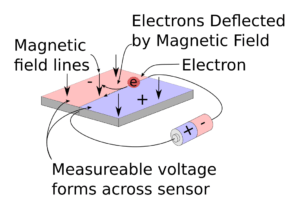The current status and classification of IMU

Generally,Inertial measurement units on the market are divided into laser IMUs, fiber optic IMUs, and MEMS IMUs. Laser IMU has high cost, high precision, and large size. It is widely used in the military. It is a technology for positioning moving objects and guiding them to their destination safely, accurately, and economically. Fiber optic IMUs are medium in cost, large in size, and relatively medium in accuracy. MEMS generally refers to micron systems of 1um to 100um, or systems with outline dimensions on the millimeter level and component sizes on the order of microns. MEMS-IMU is an inertial measurement unit based on MEMS technology. It is divided into tactical grade and navigation grade, with low precision and small size. Several high-precision, small size, light weight, low cost, and high-performance MESM IMUs have recently appeared on the market. For example, Ericco’s newly developed tactical-grade ER-MIMU03 and ER-MIMU07 and navigation-grade ER-MIMU01 and ER-MIMU05 are small in size, light in weight, low in cost, high in performance, and use high-performance north seeking. Among them, the MEMS gyroscope (ER-MG2-100), can reach 0.1°/h. The accuracy is more accurate than the lowest-precision IMUs of many large companies, and can better reflect its high performance in complex environments.

Classification of IMU:

 RLG FOG（Ericco） MEMS IMU（Ericco）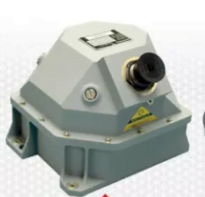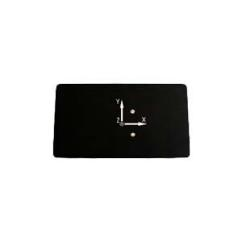Name RLG Based Navigation Grade IMU ER-FIMU-70 FOG IMU North-Seeking MEMS IMU High Accuracy North-Seeking MEMS IMU High Precision Stable-Control MEMS IMU parameter 0.0006deg/hr0.010mg0.004deg/hr0.025mg 0.05°-0.1°/hr 0.02deg/hr＜0.005°/ √h100deg/s 0.02deg/hr＜0.005°/ √h100deg/s ＜0.3deg/hr＜0.15°/ √h400deg/s

This article explains how the Inertial measurement unit works and how it works. I hope you have a general understanding of IMU. If you want to know more information, you can find useful content in “More technical questions”. If you are interested in IMU related products, you can click “Products in Articles”. If you have any questions, please leave your comments in the “Ask a Question” section below.